## New version of classical mechanics notes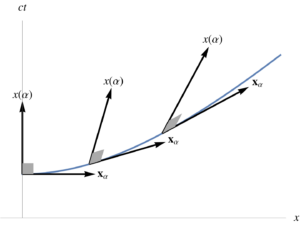I’ve posted a new version of my classical mechanics notes compilation.  This version is not yet live on amazon, but you shouldn’t buy a copy of this “book” anyways, as it is horribly rough (if you want a copy, grab the free PDF instead.)  [I am going to buy a copy so that I can continue to edit a paper copy of it, but nobody else should.]

This version includes additional background material on Space Time Algebra (STA), i.e. the geometric algebra name for the Dirac/Clifford-algebra in 3+1 dimensions.  In particular, I’ve added material on reciprocal frames, the gradient and vector derivatives, line and surface integrals and the fundamental theorem for both.  Some of the integration theory content might make sense to move to a different book, but I’ll keep it with the rest of these STA notes for now.

## More satisfying editing of classical mechanics notes.

I’ve purged about 30 pages of material related to field Lagrangian densities and Maxwell’s equation, replacing it with about 8 pages of new less incoherent material.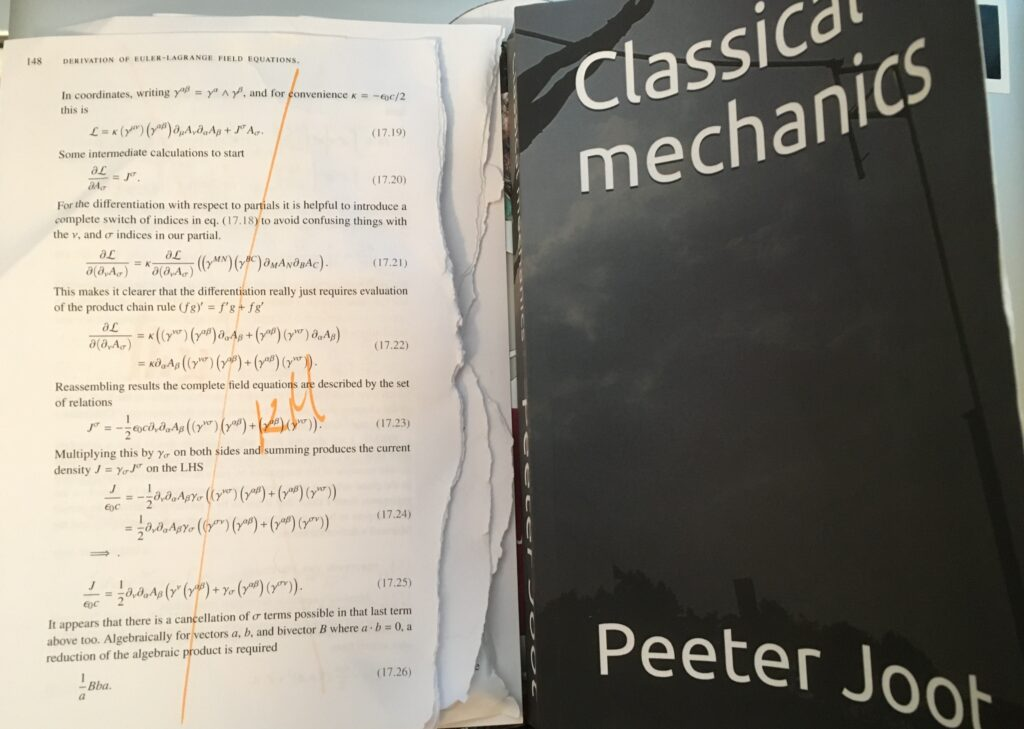As before, I’ve physically ripped out all the pages that have been replaced, which is satisfying, and makes it easier to see what is left to review.

The new version is now reduced to 333 pages, close to a 100 page reduction from the original mess.  I may print myself a new physical copy, as I’ve moved things around so much that I have to search the latex to figure out where to make changes.

## Editing a book by tearing out pages!

October 25, 2020 Uncategorized No comments ,

I’ve been editing my classical mechanics notes compilation, which doesn’t yet justify being called a book.  Here’s the editing process in action: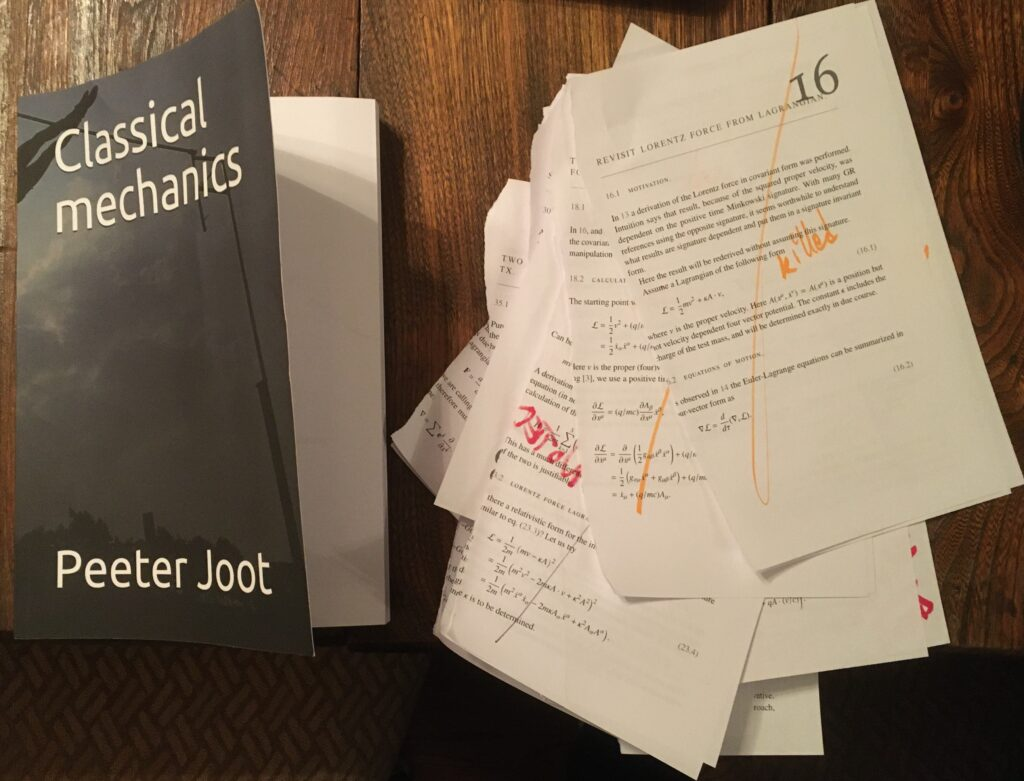I’ve purged about 120 pages, and wrote 16 new pages (covariant Lagrangian and Lorentz force material) to replace portions of some of the gutted material.  The stack beside the book is about 3/8″ thick of ripped out pages.  I ripped them out so that I could see the remainder more easily.

There’s still a lot to purge and rewrite, but I’m now down to a more manageable 347 pages, which is a good start.  Next up will be the material related to Lagrangian densities for field equations (wave equations, Maxwell’s equation, …)

## Classical mechanics notes on Amazon in paperback (but don’t buy a copy!)

October 13, 2020 math and physics play No comments ,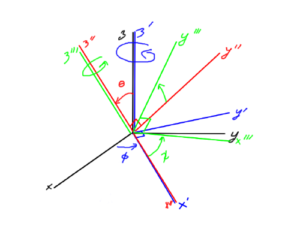I have a fairly monstrous set of classical mechanics notes that I accumulated when I was learning all about the theory of Lagrangians, Hamiltonians, and Noether’s theorem.

I also audited a few of the classes from the 2012 session of PHY354H1S, Advanced Classical Mechanics, taught by Prof. Erich Poppitz, at the University of Toronto, and have some notes and problems from those classes in this set of notes.

These notes are not self contained.  In particular, there is fairly heavy use of geometric algebra in many of the problems, with assumptions that the reader is proficient with that algebra.

These notes (436 pages, 6″x9″) are available in the following formats:

• on Amazon in paperback (black and white),
• as latex sources.

I’ve pressed the publish button on kindle-direct-publishing so that I could get a paper copy of these notes for myself.  An extremely vicious edit is required.  Until I do that editing (assuming I do), the price is set to the absolute minimum no commission price that Amazon let’s me offer (i.e. printing cost plus profit for Amazon.)  I wouldn’t actually recommend that anybody buy this in it’s current form — download the pdf if you are interested.

I’m actually toying with the idea of rewriting these notes from scratch, creating an “Advanced Classical Mechanics, with Geometric Algebra” book out of some of the ideas.  I could flush out many of the details that I explored originally, but add some actual structure and coherence to this mess of write-once-read-none junk.  Tying things to a geometric algebra theme would be the value add proposition that could distinguish things from all the other classical mechanics books in the universe.

That said, this idea would be a very tough book project (for me), as I’d have to understand all the material enough to present it in a coherent fashion.  I’d want to include and explore both Euclidean and relativistic Lagrangians, which would make the material tougher, but comprehensive.  I don’t like the idea of assuming the reader is familiar with special relativity, but the thought of me having to include a self contained introduction to that topic that isn’t complete garbage is pretty intimidating.  Especially if you consider that I’d also want to introduce STA, and help the reader understand the connections between all that material.  There’s a lot of ideas that would all have to come together!

## 4800 pages of basic physics notes for $88 USD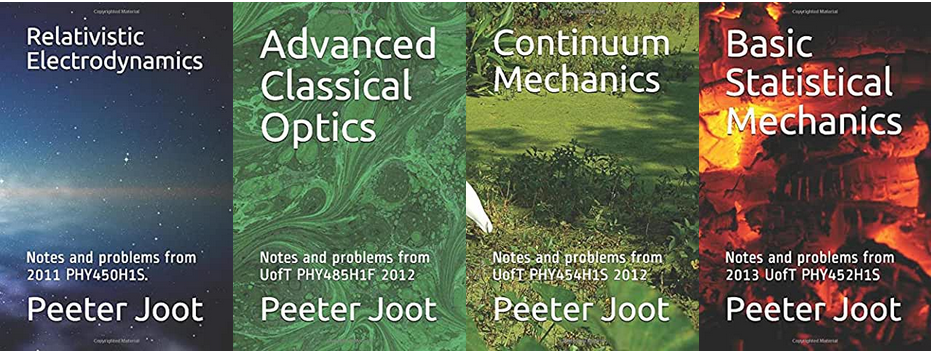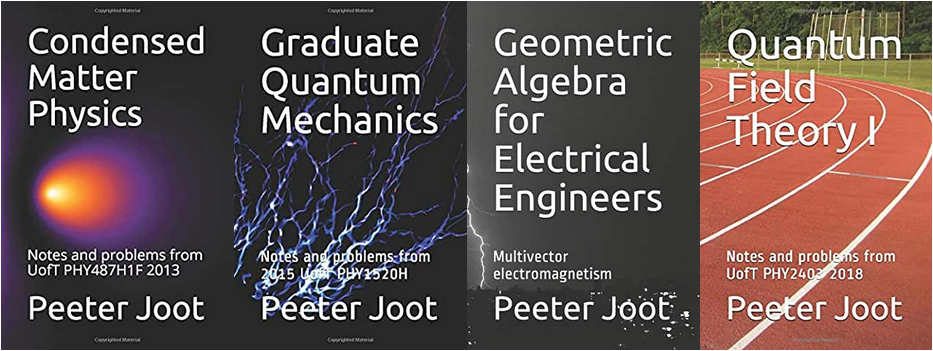Over the last 8 years I took most of the interesting 4th year undergraduate physics courses, and some graduate physics and engineering courses. Well, my notes for much of that work are now available on amazon.com (or .ca), or for free as PDF. For the bargain price of$88, leveraging the time and money that I spent, you can get very comprehensive paperback notes for these subjects.  These notes aren’t textbook quality, but generally contain detailed expositions of the subjects and many worked problems.

Here’s what’s available:

 Title Professor Year of study Format Price (USD) Pages Quantum Mechanics I: Notes and problems for UofT PHY356 2010 Prof. Vatche Deyirmenjian Fall 2010 PDF $0.00 263 Quantum Mechanics II: Notes and problems for UofT PHY456 2011 Prof. John E. Sipe Fall 2011 PDF$0.00 320 Relativistic Electrodynamics: Notes and problems from 2011 PHY450H1S Prof. Erich Poppitz Winter  2011 Black and white $11.00 387 Classical Mechanics Prof. Erich Poppitz, + self-study Winter 2012 PDF$0.00 475 Advanced Classical Optics: Notes and problems from UofT PHY485H1F 2012 Prof. Joseph H. Thywissen Fall 2012 Black and white $11.00 382 Continuum Mechanics: Notes and problems from UofT PHY454H1S 2012 Prof. Kausik S. Das Winter 2012 Black and white$10.00 358 Basic Statistical Mechanics: Notes and problems from 2013 UofT PHY452H1S Prof. Arun Paramekanti Winter 2013 Black and white $11.00 399 Condensed Matter Physics: Notes and problems from UofT PHY487H1F 2013 Prof. Stephen Julian Fall 2013 Black and white$10.00 329 Modelling of Multiphysics Systems.  Notes and problems for UofT ECE1254 Prof. Piero Triverio Fall 2014 PDF $0.00 300 Graduate Quantum Mechanics: Notes and problems from 2015 UofT PHY1520H Prof. Arun Paramekanti Winter 2015 Black and white$12.00 435 Antenna Theory: Notes and problems for UofT ECE1229 Prof G. V. Eleftheriades Winter 2015 PDF $0.00 207 Electromagnetic Theory: Notes and problems for UofT ECE1228 Prof. M. Mojahedi Fall 2016 PDF$0.00 256 Geometric Algebra for Electrical Engineers: Multivector electromagnetism self-study 2016,2017 Colour $40.00 280 Geometric Algebra for Electrical Engineers: Multivector electromagnetism self-study 2016,2017 Black and white$12.00 280 Quantum Field Theory I: Notes and problems from UofT PHY2403 2018 Prof. Erich Poppitz Fall 2018 Black and white $11.00 423 That’s 4814 pages of notes for 0-$USD 88, depending on whether you want a PDF or paper copy (if available).  My cost per page is about $4.7 CAD, factoring in total tuition costs of ~$23000 CAD (most of which was for my M.Eng), but does not factor in the opportunity cost associated with the 20% paycut (w/ a switch to 80% hours) that I also took to find the time to fit in the study.

If you compare my cost of $4.7/page for these notes to FREE –$0.024/page, then I think you would agree that my offering is a pretty good deal!  While I have built in a $1 (+/-$0.50) royalty for the book formats, the chances of me recovering my costs are infinitesimal.

A few of the courses and/or collections of notes are not worth the effort of making print ready copies, and those notes are available only in PDF form.  An exception are my notes for Multiphyiscs Modelling, which was an excellent course, and I have excellent notes for, but I’ve been asked not to make those notes available for purchase in any form (even w/ \$0 royalty.)

## PHY1520H Graduate Quantum Mechanics. Lecture 1: Lighting review. Taught by Prof. Arun Paramekanti

### Disclaimer

Peeter’s lecture notes from class. These may be incoherent and rough.

These are notes for the UofT course PHY1520, Graduate Quantum Mechanics, taught by Prof. Paramekanti, covering  chap. 1 content.

### Classical mechanics

We’ll be talking about one body physics for most of this course. In classical mechanics we can figure out the particle trajectories using both of $$(\Br, \Bp$$, where

\begin{equation}\label{eqn:qmLecture1:20}
\begin{aligned}
\ddt{\Br} &= \inv{m} \Bp \\
\end{aligned}
\end{equation}

A two dimensional phase space as sketched in fig. 1 shows the trajectory of a point particle subject to some equations of motion

### Quantum mechanics

For this lecture, we’ll work with natural units, setting

\begin{equation}\label{eqn:qmLecture1:480}
\boxed{
\Hbar = 1.
}
\end{equation}

In QM we are no longer allowed to think of position and momentum, but have to start asking about state vectors $$\ket{\Psi}$$.

We’ll consider the state vector with respect to some basis, for example, in a position basis, we write

\begin{equation}\label{eqn:qmLecture1:40}
\braket{ x }{\Psi } = \Psi(x),
\end{equation}

a complex numbered “wave function”, the probability amplitude for a particle in $$\ket{\Psi}$$ to be in the vicinity of $$x$$.

We could also consider the state in a momentum basis

\begin{equation}\label{eqn:qmLecture1:60}
\braket{ p }{\Psi } = \Psi(p),
\end{equation}

a probability amplitude with respect to momentum $$p$$.

More precisely,

\begin{equation}\label{eqn:qmLecture1:80}
\Abs{\Psi(x)}^2 dx \ge 0
\end{equation}

is the probability of finding the particle in the range $$(x, x + dx )$$. To have meaning as a probability, we require

\begin{equation}\label{eqn:qmLecture1:100}
\int_{-\infty}^\infty \Abs{\Psi(x)}^2 dx = 1.
\end{equation}

The average position can be calculated using this probability density function. For example

\begin{equation}\label{eqn:qmLecture1:120}
\expectation{x} = \int_{-\infty}^\infty \Abs{\Psi(x)}^2 x dx,
\end{equation}

or
\begin{equation}\label{eqn:qmLecture1:140}
\expectation{f(x)} = \int_{-\infty}^\infty \Abs{\Psi(x)}^2 f(x) dx.
\end{equation}

Similarly, calculation of an average of a function of momentum can be expressed as

\begin{equation}\label{eqn:qmLecture1:160}
\expectation{f(p)} = \int_{-\infty}^\infty \Abs{\Psi(p)}^2 f(p) dp.
\end{equation}

### Transformation from a position to momentum basis

We have a problem, if we which to compute an average in momentum space such as $$\expectation{p}$$, when given a wavefunction $$\Psi(x)$$.

How do we convert

\begin{equation}\label{eqn:qmLecture1:180}
\Psi(p)
\stackrel{?}{\leftrightarrow}
\Psi(x),
\end{equation}

or equivalently
\begin{equation}\label{eqn:qmLecture1:200}
\braket{p}{\Psi}
\stackrel{?}{\leftrightarrow}
\braket{x}{\Psi}.
\end{equation}

Such a conversion can be performed by virtue of an the assumption that we have a complete orthonormal basis, for which we can introduce identity operations such as

\begin{equation}\label{eqn:qmLecture1:220}
\int_{-\infty}^\infty dp \ket{p}\bra{p} = 1,
\end{equation}

or
\begin{equation}\label{eqn:qmLecture1:240}
\int_{-\infty}^\infty dx \ket{x}\bra{x} = 1
\end{equation}

Some interpretations:

1. $$\ket{x_0} \leftrightarrow \text{sits at} x = x_0$$
2. $$\braket{x}{x’} \leftrightarrow \delta(x – x’)$$
3. $$\braket{p}{p’} \leftrightarrow \delta(p – p’)$$
4. $$\braket{x}{p’} = \frac{e^{i p x}}{\sqrt{V}}$$, where $$V$$ is the volume of the box containing the particle. We’ll define the appropriate normalization for an infinite box volume later.

The delta function interpretation of the braket $$\braket{p}{p’}$$ justifies the identity operator, since we recover any state in the basis when operating with it. For example, in momentum space

\begin{equation}\label{eqn:qmLecture1:260}
\begin{aligned}
1 \ket{p}
&=
\lr{ \int_{-\infty}^\infty dp’
\ket{p’}\bra{p’} }
\ket{p} \\
&=
\int_{-\infty}^\infty dp’
\ket{p’}
\braket{p’}{p} \\
&=
\int_{-\infty}^\infty dp’
\ket{p’}
\delta(p – p’) \\
&=
\ket{p}.
\end{aligned}
\end{equation}

This also the determination of an integral operator representation for the delta function

\begin{equation}\label{eqn:qmLecture1:500}
\begin{aligned}
\delta(x – x’)
&=
\braket{x}{x’} \\
&=
\int dp \braket{x}{p} \braket{p}{x’} \\
&=
\inv{V} \int dp e^{i p x} e^{-i p x’},
\end{aligned}
\end{equation}

or

\begin{equation}\label{eqn:qmLecture1:520}
\delta(x – x’)
=
\inv{V} \int dp e^{i p (x- x’)}.
\end{equation}

Here we used the fact that $$\braket{p}{x} = \braket{x}{p}^\conj$$.

FIXME: do we have a justification for that conjugation with what was defined here so far?

The conversion from a position basis to momentum space is now possible

\begin{equation}\label{eqn:qmLecture1:280}
\begin{aligned}
\braket{p}{\Psi}
&= \Psi(p) \\
&= \int_{-\infty}^\infty \braket{p}{x} \braket{x}{\Psi} dx \\
&= \int_{-\infty}^\infty \frac{e^{-ip x}}{\sqrt{V}} \Psi(x) dx.
\end{aligned}
\end{equation}

The momentum space to position space conversion can be written as

\begin{equation}\label{eqn:qmLecture1:300}
\Psi(x)
= \int_{-\infty}^\infty \frac{e^{ip x}}{\sqrt{V}} \Psi(p) dp.
\end{equation}

Now we can go back and figure out the an expectation

\begin{equation}\label{eqn:qmLecture1:320}
\begin{aligned}
\expectation{p}
&=
\int \Psi^\conj(p) \Psi(p) p d p \\
&=
\int dp
\lr{
\int_{-\infty}^\infty \frac{e^{ip x}}{\sqrt{V}} \Psi^\conj(x) dx
}
\lr{
\int_{-\infty}^\infty \frac{e^{-ip x’}}{\sqrt{V}} \Psi(x’) dx’
}
p \\
&=\int dp dx dx’
\Psi^\conj(x)
\inv{V} e^{ip (x-x’)} \Psi(x’) p \\
&=
\int dp dx dx’
\Psi^\conj(x)
\inv{V} \lr{ -i\PD{x}{e^{ip (x-x’)}} }\Psi(x’) \\
&=
\int dp dx
\Psi^\conj(x) \lr{ -i \PD{x}{} }
\inv{V} \int dx’ e^{ip (x-x’)} \Psi(x’) \\
&=
\int dx
\Psi^\conj(x) \lr{ -i \PD{x}{} }
\int dx’ \lr{ \inv{V} \int dp e^{ip (x-x’)} } \Psi(x’) \\
&=
\int dx
\Psi^\conj(x) \lr{ -i \PD{x}{} }
\int dx’ \delta(x – x’) \Psi(x’) \\
&=
\int dx
\Psi^\conj(x) \lr{ -i \PD{x}{} }
\Psi(x)
\end{aligned}
\end{equation}

Here we’ve essentially calculated the position space representation of the momentum operator, allowing identifications of the following form

\begin{equation}\label{eqn:qmLecture1:380}
p \leftrightarrow -i \PD{x}{}
\end{equation}
\begin{equation}\label{eqn:qmLecture1:400}
p^2 \leftrightarrow – \PDSq{x}{}.
\end{equation}

### Alternate starting point.

Most of the above results followed from the claim that $$\braket{x}{p} = e^{i p x}$$. Note that this position space representation of the momentum operator can also be taken as the starting point. Given that, the exponential representation of the position-momentum braket follows

\begin{equation}\label{eqn:qmLecture1:540}
\bra{x} P \ket{p}
=
-i \Hbar \PD{x}{} \braket{x}{p},
\end{equation}

but $$\bra{x} P \ket{p} = p \braket{x}{p}$$, providing a differential equation for $$\braket{x}{p}$$

\begin{equation}\label{eqn:qmLecture1:560}
p \braket{x}{p} = -i \Hbar \PD{x}{} \braket{x}{p},
\end{equation}

with solution

\begin{equation}\label{eqn:qmLecture1:580}
i p x/\Hbar = \ln \braket{x}{p} + \text{const},
\end{equation}

or
\begin{equation}\label{eqn:qmLecture1:600}
\braket{x}{p} \propto e^{i p x/\Hbar}.
\end{equation}

### Matrix interpretation

1. Ket’s $$\ket{\Psi} \leftrightarrow \text{column vector}$$
2. Bra’s $$\bra{\Psi} \leftrightarrow {(\text{row vector})}^\conj$$
3. Operators $$\leftrightarrow$$ matrices that act on vectors.

\begin{equation}\label{eqn:qmLecture1:420}
\hat{p} \ket{\Psi} \rightarrow \ket{\Psi’}
\end{equation}

### Time evolution

For a state subject to the equations of motion given by the Hamiltonian operator $$\hat{H}$$

\begin{equation}\label{eqn:qmLecture1:440}
i \PD{t}{} \ket{\Psi} = \hat{H} \ket{\Psi},
\end{equation}

the time evolution is given by
\begin{equation}\label{eqn:qmLecture1:460}
\ket{\Psi(t)} = e^{-i \hat{H} t} \ket{\Psi(0)}.
\end{equation}

### Incomplete information

We’ll need to introduce the concept of Density matrices. This will bring us to concepts like entanglement.

# References

 Jun John Sakurai and Jim J Napolitano. Modern quantum mechanics. Pearson Higher Ed, 2014.